# Non-differentiable function

(diff) ← Older revision | Latest revision (diff) | Newer revision → (diff)

A function that does not have a differential. In the case of functions of one variable it is a function that does not have a finite derivative. For example, the function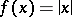is not differentiable at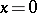, though it is differentiable at that point from the left and from the right (i.e. it has finite left and right derivatives at that point). The continuous function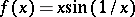if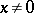andis not only non-differentiable at, it has neither left nor right (and neither finite nor infinite) derivatives at that point.

The first examples of functions continuous on the entire real line but having no finite derivative at any point were constructed by B. Bolzano in 1830 (published in 1930) and by K. Weierstrass in 1860 (published in 1872). Weierstrass' function is the sum of the serieswhere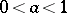,is an odd natural number and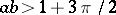. A simpler example, based on the same idea, in which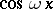is replaced by a simpler periodic function — a polygonal line — was constructed by B.L. van der Waerden. Letbe the function defined for realas the absolute value of the difference betweenand the nearest integer. This function is linear on every interval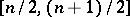, whereis an integer; it is continuous and periodic with period 1. Let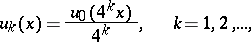then van der Waerden's function is defined byThis function is continuous on the entire real line but does not have a finite derivative at any point. The first three partial sums of the series are shown in the figure.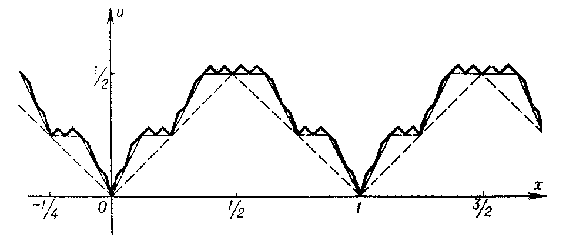Figure: n067010a

For functions of more than one variable, differentiability at a point is not equivalent to the existence of the partial derivatives at the point; there are examples of non-differentiable functions that have partial derivatives. For example, the function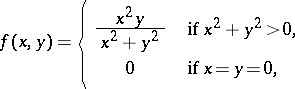is continuous at all points of the plane and has partial derivatives everywhere but it is not differentiable at.

S. Banach proved that "most" continuous functions are nowhere differentiable. Specifically, he showed that ifdenotes the space of all continuous real-valued functions on the unit interval, equipped with the uniform metric (sup norm), then the set of members ofthat have a finite right-hand derivative at some point of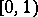is of the first Baire category (cf. Baire classes) in the complete metric space. Proof of this fact and of the nowhere differentiability of Weierstrass' example cited above can be found in [a1]. A proof that van der Waerden's example has the stated properties can be found in [a2].